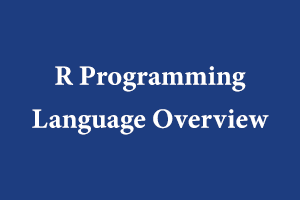Author - StudySection Post Views - 88 views# R Programming Language Overview

R is a programming language that is used for statistical and visualization analysis. It is also used for reporting. We use this language for data analysis, which gives us results in the form of graphs, pie charts, etc. R is an interpreted programming language. It is a simple and well-developed language that uses loops, functions, conditions, etc. For calculations, it uses arrays, matrix, list, and vectors. It provides a graphical representation of the data. It also processes large datasets.
The basic syntax is given below:

• In the R language, there is no need to give data type to a variable. Directly, give value to the variable.
• Following is the basic syntax of how to print string in R language:
```test<-” Good Morning”; print(test); o/p:  “Good Morning”```
• To comment code in R language # is used.
`Eg: #test<-” Good Morning”;`

### DATA TYPES: To check the data type of the variable class() is used.

1. Logical: It is a type of data type used for True or False value.
```Eg: test<-TRUE print(class(test)) o/p:  "logical"```
2. Numeric: It is used for numerical data or numbers.
```Eg: test<-8 print(class(test)) o/p:  "numeric```"
3. Complex: It is used for complex data that contains iota values.
```Eg: test<-1+2i print(class(test)) o/p:  "complex"```
4. Character: It is used for all the characters. For strings, it belongs to the character datatype.
```Eg: test<-”HELLO” print(class(test)) o/p:  "character"```
5. Raw: It is used for the raw character.
```Eg: test<-charToRaw(”HELLO”) print(class(test)) o/p:  "raw"```

### R-Objects: These objects use data types as described above.

1. Vectors: Most basic R-object is vectors. Function c() is used for the vectors.
```Eg: test <- c('bmw','audi',’maruti’) print(test) o/p:  "bmw" "audi" "maruti" # To find class of the vector print(class(test))  "character"```
2. Lists: It contains different types of elements in it. It can be numbers, characters, functions, even other lists, etc.
```Eg: l1 <- list(c(1,2,3),1.5,3.8) print(l1) o/p: []  1 2 3 []  1.5 3.8```
3. Matrices: Matrix is a 2-D rectangular data set. It is created using vectors in matrix().
```Eg: m = matrix( c('1','2','3','4','5','6'), nrow = 2, ncol = 3, byrow = TRUE) #Where nrow is for number of rows, ncol is for number of columns. print(m) o/p: [,1] [,2] [,3] [1,] "1" "2" "3" [2,] "4" "5" "6"```
4. Arrays: Unlike matrices, Arrays can have any number of dimensions. ‘dim’ attribute is used to create the required number of dimensions.
```Eg: arr <- array(c('e1','e2'),dim = c(3,3,2)) # Set dimension of 3 * 3 and the number of arrays will be 2. print(arr) o/p: , , 1 [,1] [,2] [,3] [1,] "e1" "e2" "e1" [2,] "e2" "e1" "e2" [3,] "e1" "e2" "e1" ,levels [,1] [,2] [,3] [1,] "e2" "e1" "e2" [2,] "e1" "e2" "e1" [3,] "e2" "e1" "e2"```
5. Factors: They are created using vectors. factor() is used to create the factors and nlevels() gives the count of levels.
```Eg: f1<-c('1','1','2','3','3','2','1') # Create a factor object. fo <- factor(f1) # Print the factor. print(f1) print(nlevels(fo)) o/p:  1 1 2 3 3 2 1 Levels: 1 2 3  3```
6. Data Frames: It is used for tabular data objects. Every column can contain different types of data. It is created with a list of vectors having an equal length. Data frames are created using data.frame().
```Eg: vehicle <- data.frame( car = c("c1", "c2","c3"), bike = c(1, 2, 3) ) print(vehicle) o/p: car bike 1 c1 1 2 c2 2 3 c3 3```

Conclusion: R language is one of the most widely used Statistical programming languages. This language is used by data scientists.

jQuery presents a tree-like structure of all the elements on a webpage simplifying the syntax and further manipulating such elements. The jQuery Certification Exam by StudySection will secure your fundamental knowledge and a basic understanding of jQuery as an asset to improve your skills.